## Saturday, August 4, 2007

### Irodov Problem 1.61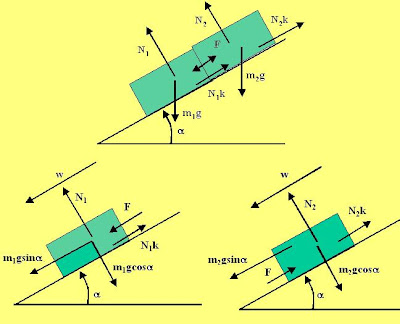(a) The force diagram of the problem is depicted in the picture beside. It is easier to solve the problem if we resolve all forces along the directions parallel and perpendicular to the inclined plane. This is shown for each of the two bodies separately in the figure.

Forces acting on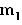:
Let us first consider the direction perpendicular to the inclined plane. There are only two forces acting in this direction - the normal reactionfrom the surface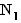and the component of gravity that acts on the mass,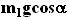. There is no acceleration for the mass in this direction and hence we have,Now, let us consider the forces along the direction of the inclined plane. There are three forces acting in this direction - i) the component of gravity trying to accelerate the mass down, ii) the force of friction opposing the direction of motionand iii) the normal reaction from body 2 to body 1, F pushing downwards along the inclined plane. Suppose that the mass accelerates downwards with an acceleration of w along the inclined plane. We have,Forces on:
There are two forces acting in the perpendicular direction to the inclined plane - the normal reaction from the inclined planeand the component of force of gravityin a direction perpendicular to the inclined plane. There is no acceleration for body 2 along this direction. Thus, we have,There are three forces acting in the horizontal direction, i) the component of gravity along the inclined plane, ii) the normal reaction from body 1 to body 2 pushing body 2 up the inclined plane F and, iii) the force of friction that opposes the motion of body 2 given by,. The body also accelerates at a rate w along this direction down the inclined plane. Thus, we have,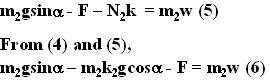Now we can solve for the unknowns F and w. Adding (6) and (3),Now we can substitute (7) in either (3) or (6) to obtain,(b) Strictly speaking friction coefficient during motion and during the time when the body is static are usually different values - the friction coefficient in the static position is usually high. In this problem however, both these values are assumed to be the same. The friction force constantly adjusts itself and provide equal and opposite force to oppose motion until the maximum friction it can provide (normal reaction times the coefficient of friction) is reached. Thus, all have to do is set w=0 in (7) and so we have,#### 1 comment:Anonymous said...

Thanx a lot.